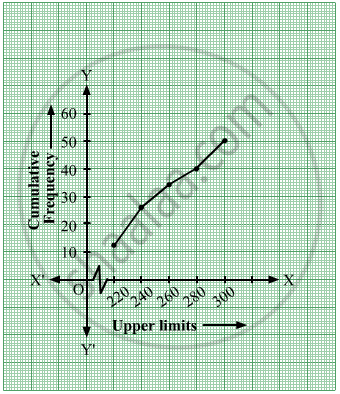# The Following Distribution Gives the Daily Income of 50 Workers of a Factory. - Mathematics

Sum

The following distribution gives the daily income of 50 workers of a factory.

 Daily income (in ₹) 200-220 220-240 240-260 260-280 280-300 Number of workers 12 14 8 6 10

Convert the distribution above to a 'less than type' cumulative frequency distribution and draw its ogive.

#### Solution

The less than type cumulative frequency distribution table will be as follows:

 Daily income(in ₹) Number of Workers Daily Income Less than Cumulative Frequency 200 - 220 12 220 12 220 - 240 14 240 12 + 14 = 26 240 - 260 8 260 26 + 8 = 34 260 - 280 6 280 34 + 6 = 40 280 - 300 10 300 40 + 10 = 50

The ogive thus formed will be​Concept: Graphs of Linear Equations
Is there an error in this question or solution?